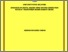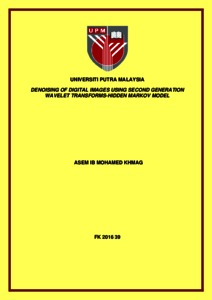# Denoising of digital images using second generation wavelet transforms-hidden markov model

## Citation

Khmag, Asem Ib Mohamed (2016) Denoising of digital images using second generation wavelet transforms-hidden markov model. Doctoral thesis, Universiti Putra Malaysia.

## Abstract

The digital images are defined as digital signals come across with many kinds of difficult scenarios during transmission and acquisition. One of the main problems in the scientific digital world is the noise. For example, amendments due to additive white Gaussian noise (AWGN) or the multiplicative (speckle) in some cases that can be resulted from poor quality image acquisition. The main aim of an image denoising technique is to minimize the level of noise, and protect the fine details of the image as much as possible. Wavelet denoising has the superior reputation to remove the additive noise while preserving the signal features, nevertheless of its frequency content. In this regard, the second-generation wavelets (SGWs) that employ the properties of sparsity and multiresolution from discrete wavelet transformation (DWT) is used in noise reduction. The aim of this thesis is to restore a high quality image from the noisy counterpart where the natural images mostly suffered from AWGN. In this issue, a newly developed algorithm based on second-generation wavelet transformation using semi-soft thresholding is introduced. In order to increase the robustness of the proposed algorithm, the level of noise in digital image can be estimated if the noise standard deviation σ is unknown. This estimation can be done by exploiting one of the features of SGWs. Moreover, to capture the dependency between a pixel and its neighbours on the wavelet transform, hidden Markov model (HMM) is used. The HMM also allows the hidden states to connect to each other to capture the dependencies among the coefficients in wavelet domain. Due to the lack of translation invariance in the wavelet basis function, some artifacts may appear after applying the denoising algorithm. Cycle spinning idea (for range of shift operations) is implemented in order to enhance the quality of the denoised estimates, and minimize the Gibbs phenomenon disturbing artifacts that are often existing in wavelet-based image reconstruction and denoising.The main steps in the proposed denoised algorithm are: firstly, apply the second–generation wavelet transformation on the noisy image. Then perform hierarchically,point-wise adaptive thresholding on the wavelet coefficients. Once the wavelet coefficients are modified, the estimation of the wavelet coefficients using HMM is determined. Finally, the inverse process can be applied to the wavelet coefficients to restore it back to the original form and attain the denoised image. After applying the denoising algorithm on the contaminated image, the cycling spinning algorithm is applied to increase the visual quality of the restored image. Furthermore, to evaluate the suggested algorithm, two kinds of assessment scales are conducted; subjective and objective scales. Firstly, quantitative comparison that represents the objective scale is used in the proposed algorithm to evaluate the denoised images. It contains assessment measurements such as: peak signal to noise ratio (PSNR), mean squared error (MSE),structure similarity index (SSIM), and finally the image quality index (Q-index).Secondly, subjective scale, good measureable results do not assurance high visual quality of the denoised images. So in real applications, the visual quality is still an important metric. According to that, the visual comparison in subjective analysis is used. The denoised images subjected to a poll where people were asked to pick the two least noisy images, and rank them as first and second choice. The images that are chosen to be tested in this study are: Lena, Barbara, Baboon, Boat, F16 and Peppers.The suggested algorithm (SGWs-HMM) outperformed the best state-of-the-art denoising algorithms in terms of quantitative measurements and design simplicity in most of the time. Mathematically, in PSNR, the improvement margin of SGWs-HMM was in range from 0.6dB up to 5.6dB compared with different denoising algorithms under investigation, and in SSIM it showed (~0.5-0.72) higher than HMM and Block matching 3D (BM3D) algorithms in different tested images. As a conclusion, the digital image denoising technique that is proposed in this study can enhance and improve the noisy images both qualitatively and quantitatively. The proposed algorithm is designed in order to tackle many limitations of the existing algorithms such as complexity load, inability to be universal, vulnerability to severe image degradations especially in high noise levels, etc.Preview
Text
FK 2016 39 IR.pdfView Item